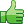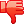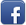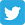Posted on 16th Feb 2014 07:03 pm by admin

Ok so I just learned quickly about lists, so I have a not too hard project I think, but am having a few issues:

Here is the project. Create a lovely polynomial calculator, that takes the equation from the user, and a number for x.
Then solve it, but I have to use lists. As well as that I have to printout the equation with x at the end. And I will also have to have to add a method for finding the derivative of the equation.

I'll worry about the printout and the derivative at the end, except I have a problem right now... I'll show my code first

Here is the lovely main
Code: import java.util.*;public class PolyDriver { public static void main(String[] args) { boolean isrunning = true; String userinput="no"; String no="no"; String yes="yes"; double whatisx=0; Scanner scan = new Scanner(System.in); System.out.println("What is X?"); whatisx =scan.nextDouble(); while(userinput.equals(no)) { System.out.println("Enter the first integer"); double d = scan.nextDouble(); System.out.println("Enter the exponent"); int e = scan.nextInt(); Poly m = new Poly(d, e, whatisx); System.out.println("Done?"); String j = scan.next(); if(j.equals(yes)) { System.exit(0); } } // System.out.println("Enter the number for x"); // Scanner scan = new Scanner(System.in); }}

Then here is the Poly class

Code: import java.util.*;/* * To change this template, choose Tools | Templates * and open the template in the editor. *//** * * @author JigglyWiggly-laptop */public class Poly { double integer=0; int exponent=0; double variable=0; double vrz=0; double whatisx=0; LinkedList linkedlist = new LinkedList(); public Poly(double d, int e, double x) { integer=d; exponent=e; whatisx=x; calculations(); // calculations(); } public double calculations() { double v = Math.pow(whatisx, exponent); double j = v*integer; linkedlist.add(j); printout(); return j; } public double printout() { for(int i=0; iWhen I run it:
Code: run:What is X?2Enter the first integer2Enter the exponent2---18.0Done?noEnter the first integer2Enter the exponent2---18.0Done?noEnter the first integer2Enter the exponent2---18.0Done? Why does it not make the list bigger? And the numbers should be adding up. Any ideas?

(Also ignore the funky way of getting the user input, this is the way we were told to do so)

31844##### Other forums

Oracle Connectivity
Hi Every One,

Can we access SAP from oracle database.If it possible then please spec

multipart emiail forms
Hi All,

I am new to the boards and I've been working on a form (which is rather massive, imo

How to submit a form to the same page?
I have a table containing information about books in my library and this table has the following col

PHP - HTML
Could anyone give me some GOOD sample links for php - html email tutorial.

Thanks!

Find occurences of unicode characters in string
I need to prohibit filenames with everything but English characters and numbers but regexp and strin